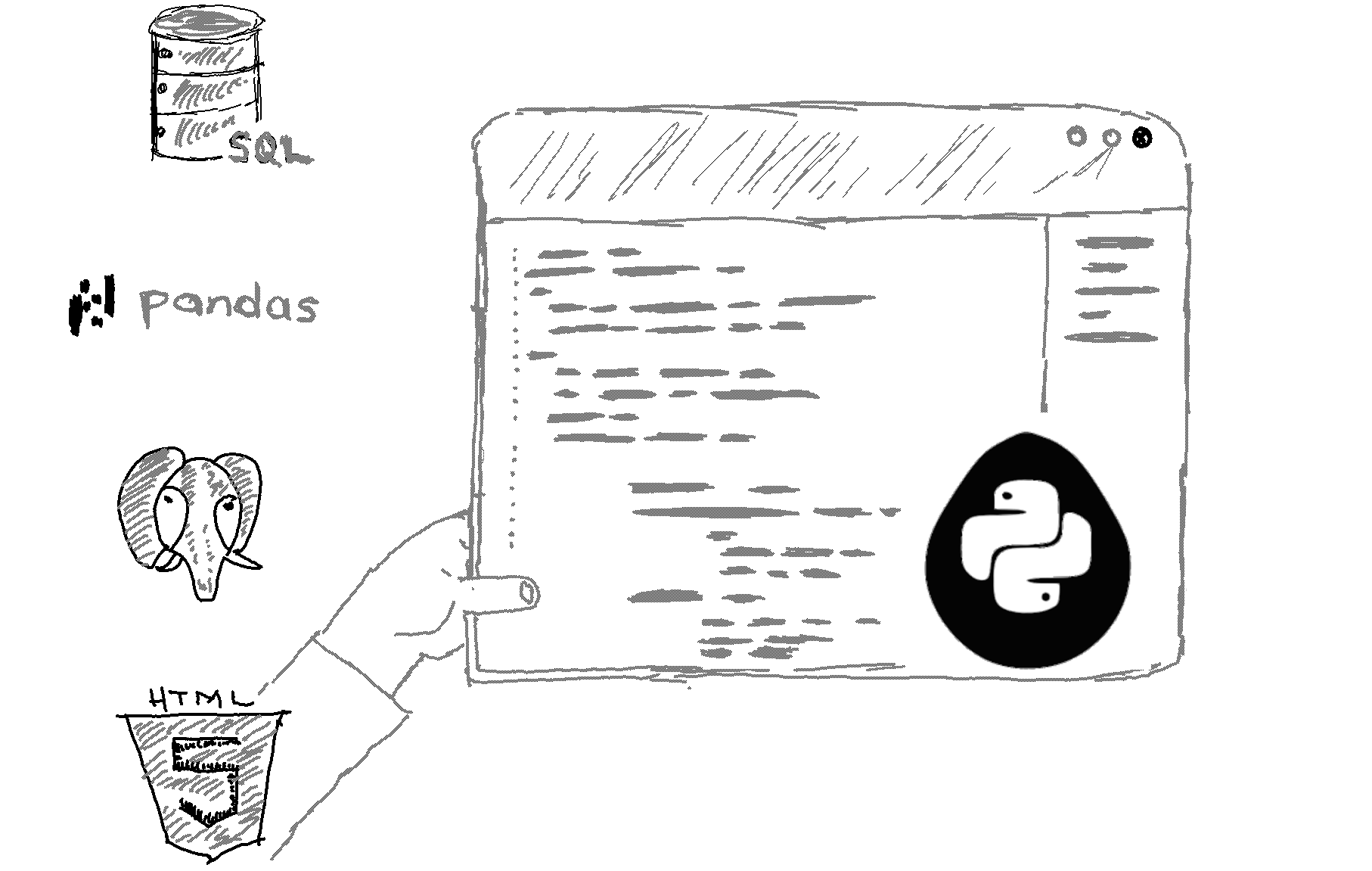# Here is how to measure elapsed time in Python in Python.

```import time

# Start timer
start_time = time.time()

# Code to be timed
# ...

# End timer
end_time = time.time()

# Calculate elapsed time
elapsed_time = end_time - start_time
print("Elapsed time: ", elapsed_time) ```

Output

Explanation

In Python, you can use the time module to measure elapsed time. You can use the time() function to get the current time in seconds since the epoch (January 1, 1970, at 00:00:00 UTC). Then, you can subtract the start time from the end time to get the elapsed time.

Alternatively, you can use the perf_counter() or monotonic() function to measure the time, that depends on the specific use case:

```import time

# Start timer
start_time = time.perf_counter()

# Code to be timed
# ...

# End timer
end_time = time.perf_counter()

# Calculate elapsed time
elapsed_time = end_time - start_time
print("Elapsed time: ", elapsed_time)
```

Related HowTos
##### Recommended Course

Learn Flask development and learn to build cool apps with our premium Python course on Udemy.